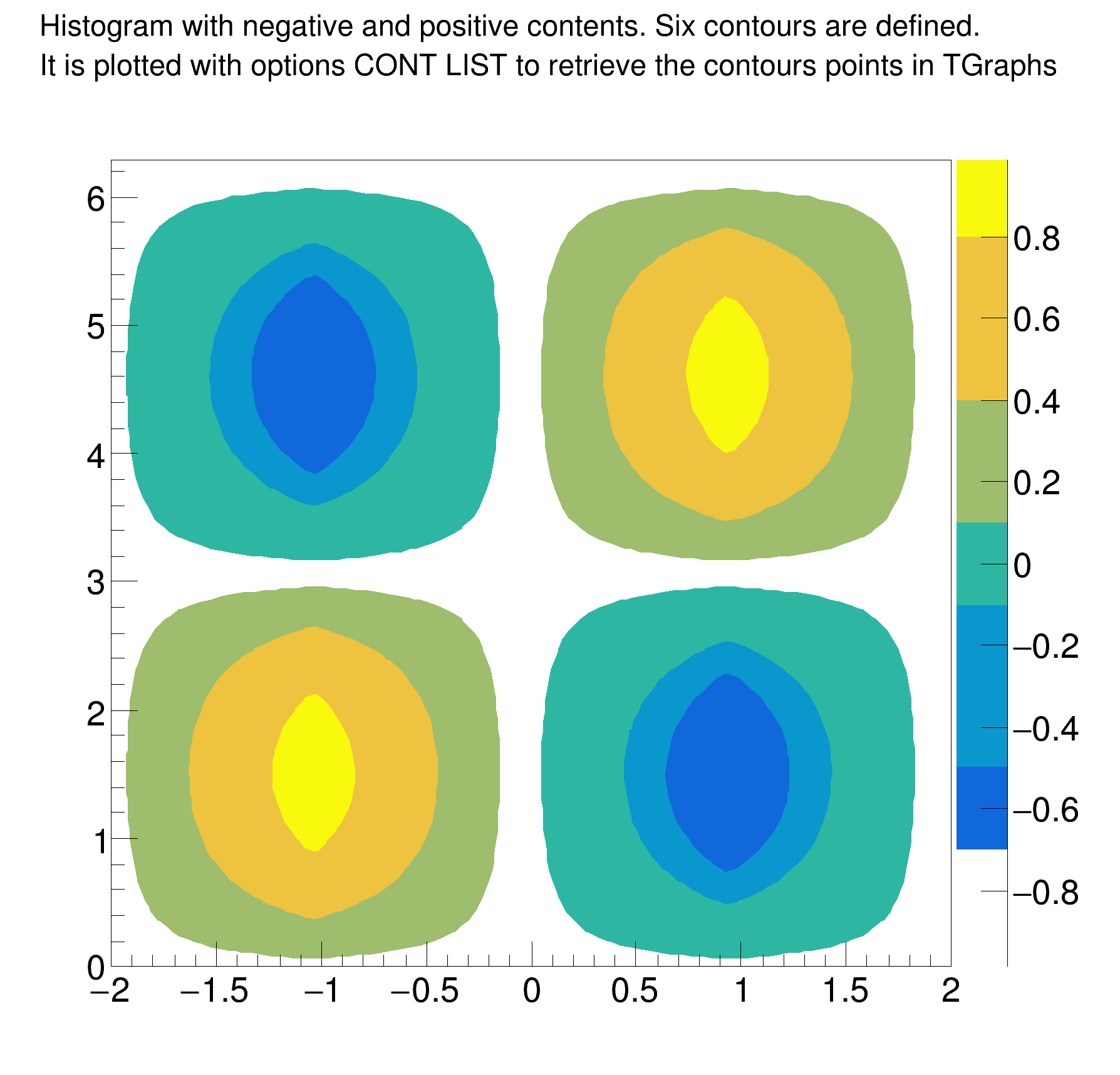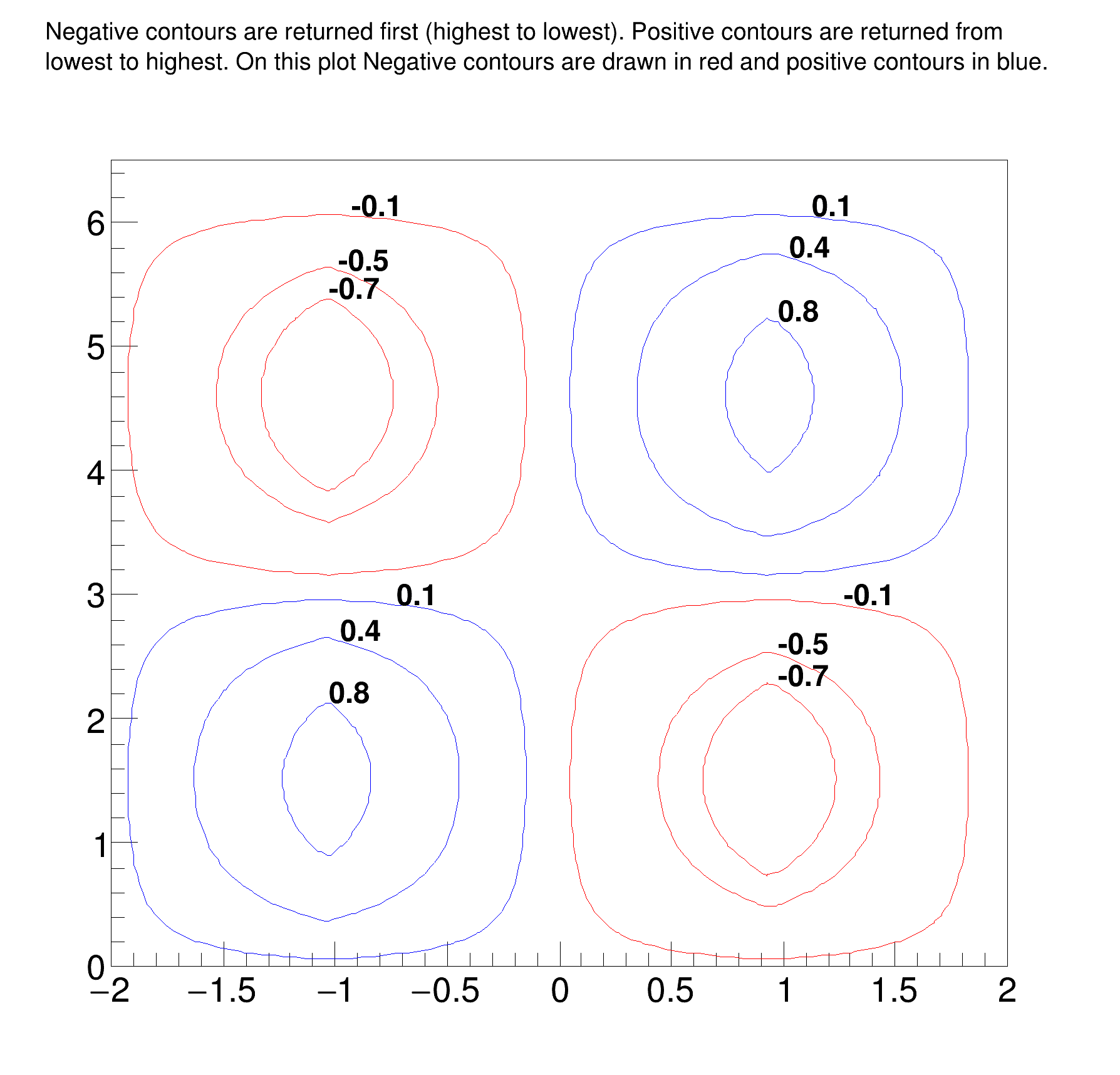ROOT   Reference GuideContourList.C File Reference

## Detailed Description

#### Image produced by .x ContourList.C

The contours values are drawn next to each contour.#### Output produced by .x ContourList.C

It shows that 6 contours and 12 graphs were found.

TotalConts = 6
Contour 0 has 2 Graphs
Contour 1 has 2 Graphs
Contour 2 has 2 Graphs
Contour 3 has 2 Graphs
Contour 4 has 2 Graphs
Contour 5 has 2 Graphs
Z-Level Passed in as: Z = -0.100000
Graph: 1 -- 147 Elements
Graph: 2 -- 147 Elements
Z-Level Passed in as: Z = -0.500000
Graph: 3 -- 93 Elements
Graph: 4 -- 93 Elements
Z-Level Passed in as: Z = -0.700000
Graph: 5 -- 65 Elements
Graph: 6 -- 65 Elements
Z-Level Passed in as: Z = 0.100000
Graph: 7 -- 147 Elements
Graph: 8 -- 147 Elements
Z-Level Passed in as: Z = 0.400000
Graph: 9 -- 107 Elements
Graph: 10 -- 107 Elements
Z-Level Passed in as: Z = 0.800000
Graph: 11 -- 49 Elements
Graph: 12 -- 49 Elements
Extracted 6 Contours and 12 Graphs
(TCanvas *) 0x55a8be37d030

#### ContourList.C

Double_t SawTooth(Double_t x, Double_t WaveLen);
TCanvas *ContourList(){
const Double_t PI = TMath::Pi();
TCanvas* c = new TCanvas("c","Contour List",0,0,600,600);
c->SetRightMargin(0.15);
c->SetTopMargin(0.15);
Int_t i, j;
Int_t nZsamples = 80;
Int_t nPhiSamples = 80;
Double_t HofZwavelength = 4.0; // 4 meters
Double_t dZ = HofZwavelength/(Double_t)(nZsamples - 1);
Double_t dPhi = 2*PI/(Double_t)(nPhiSamples - 1);
TArrayD z(nZsamples);
TArrayD HofZ(nZsamples);
TArrayD phi(nPhiSamples);
TArrayD FofPhi(nPhiSamples);
// Discretized Z and Phi Values
for ( i = 0; i < nZsamples; i++) {
z[i] = (i)*dZ - HofZwavelength/2.0;
HofZ[i] = SawTooth(z[i], HofZwavelength);
}
for(Int_t i=0; i < nPhiSamples; i++){
phi[i] = (i)*dPhi;
FofPhi[i] = sin(phi[i]);
}
// Create Histogram
TH2D *HistStreamFn = new TH2D("HstreamFn",
"#splitline{Histogram with negative and positive contents. Six contours are defined.}{It is plotted with options CONT LIST to retrieve the contours points in TGraphs}",
nZsamples, z, z[nZsamples-1], nPhiSamples, phi, phi[nPhiSamples-1]);
for (Int_t i = 0; i < nZsamples; i++) {
for(Int_t j = 0; j < nPhiSamples; j++){
HistStreamFn->SetBinContent(i,j, HofZ[i]*FofPhi[j]);
}
}
gStyle->SetTitleW(0.99);
gStyle->SetTitleH(0.08);
Double_t contours;
contours = -0.7;
contours = -0.5;
contours = -0.1;
contours = 0.1;
contours = 0.4;
contours = 0.8;
HistStreamFn->SetContour(6, contours);
// Draw contours as filled regions, and Save points
HistStreamFn->Draw("CONT Z LIST");
c->Update(); // Needed to force the plotting and retrieve the contours in TGraphs
// Get Contours
TObjArray *conts = (TObjArray*)gROOT->GetListOfSpecials()->FindObject("contours");
TList* contLevel = NULL;
TGraph* curv = NULL;
TGraph* gc = NULL;
Int_t nGraphs = 0;
Int_t TotalConts = 0;
if (conts == NULL){
printf("*** No Contours Were Extracted!\n");
TotalConts = 0;
return 0;
} else {
TotalConts = conts->GetSize();
}
printf("TotalConts = %d\n", TotalConts);
for(i = 0; i < TotalConts; i++){
contLevel = (TList*)conts->At(i);
printf("Contour %d has %d Graphs\n", i, contLevel->GetSize());
nGraphs += contLevel->GetSize();
}
nGraphs = 0;
TCanvas* c1 = new TCanvas("c1","Contour List",610,0,600,600);
c1->SetTopMargin(0.15);
TH2F *hr = new TH2F("hr",
"#splitline{Negative contours are returned first (highest to lowest). Positive contours are returned from}{lowest to highest. On this plot Negative contours are drawn in red and positive contours in blue.}",
2, -2, 2, 2, 0, 6.5);
hr->Draw();
Double_t xval0, yval0, zval0;
l.SetTextSize(0.03);
char val;
for(i = 0; i < TotalConts; i++){
contLevel = (TList*)conts->At(i);
if (i<3) zval0 = contours[2-i];
else zval0 = contours[i];
printf("Z-Level Passed in as: Z = %f\n", zval0);
// Get first graph from list on curves on this level
curv = (TGraph*)contLevel->First();
for(j = 0; j < contLevel->GetSize(); j++){
curv->GetPoint(0, xval0, yval0);
if (zval0<0) curv->SetLineColor(kRed);
if (zval0>0) curv->SetLineColor(kBlue);
nGraphs ++;
printf("\tGraph: %d -- %d Elements\n", nGraphs,curv->GetN());
// Draw clones of the graphs to avoid deletions in case the 1st
gc = (TGraph*)curv->Clone();
gc->Draw("C");
sprintf(val,"%g",zval0);
l.DrawLatex(xval0,yval0,val);
curv = (TGraph*)contLevel->After(curv); // Get Next graph
}
}
c1->Update();
printf("\n\n\tExtracted %d Contours and %d Graphs \n", TotalConts, nGraphs );
return c1;
}
Double_t SawTooth(Double_t x, Double_t WaveLen){
// This function is specific to a sawtooth function with period
// WaveLen, symmetric about x = 0, and with amplitude = 1. Each segment
// is 1/4 of the wavelength.
//
// |
// /\ |
// / \ |
// / \ |
// / \
// /--------\--------/------------
// |\ /
// | \ /
// | \ /
// | \/
//
if ( (x < -WaveLen/2) || (x > WaveLen/2)) y = -99999999; // Error X out of bounds
if (x <= -WaveLen/4) {
y = x + 2.0;
} else if ((x > -WaveLen/4) && (x <= WaveLen/4)) {
y = -x ;
} else if (( x > WaveLen/4) && (x <= WaveLen/2)) {
y = x - 2.0;
}
return y;
}
#define c(i)
Definition: RSha256.hxx:101
int Int_t
Definition: RtypesCore.h:45
double Double_t
Definition: RtypesCore.h:59
@ kRed
Definition: Rtypes.h:66
@ kBlue
Definition: Rtypes.h:66
#define PI
double sin(double)
#define gROOT
Definition: TROOT.h:406
R__EXTERN TStyle * gStyle
Definition: TStyle.h:412
Array of doubles (64 bits per element).
Definition: TArrayD.h:27
virtual void SetLineColor(Color_t lcolor)
Set the line color.
Definition: TAttLine.h:40
The Canvas class.
Definition: TCanvas.h:23
virtual Int_t GetSize() const
Return the capacity of the collection, i.e.
Definition: TCollection.h:182
A TGraph is an object made of two arrays X and Y with npoints each.
Definition: TGraph.h:41
Int_t GetN() const
Definition: TGraph.h:124
virtual void Draw(Option_t *chopt="")
Draw this graph with its current attributes.
Definition: TGraph.cxx:769
virtual Int_t GetPoint(Int_t i, Double_t &x, Double_t &y) const
Get x and y values for point number i.
Definition: TGraph.cxx:1607
virtual void SetContour(Int_t nlevels, const Double_t *levels=0)
Set the number and values of contour levels.
Definition: TH1.cxx:8331
virtual void Draw(Option_t *option="")
Draw this histogram with options.
Definition: TH1.cxx:3073
2-D histogram with a double per channel (see TH1 documentation)}
Definition: TH2.h:292
2-D histogram with a float per channel (see TH1 documentation)}
Definition: TH2.h:251
virtual void SetBinContent(Int_t bin, Double_t content)
Set bin content.
Definition: TH2.cxx:2507
To draw Mathematical Formula.
Definition: TLatex.h:18
Definition: TList.h:44
virtual TObject * After(const TObject *obj) const
Returns the object after object obj.
Definition: TList.cxx:330
virtual TObject * First() const
Return the first object in the list. Returns 0 when list is empty.
Definition: TList.cxx:659
virtual TObject * Clone(const char *newname="") const
Make a clone of an object using the Streamer facility.
Definition: TNamed.cxx:74
An array of TObjects.
Definition: TObjArray.h:37
TObject * At(Int_t idx) const
Definition: TObjArray.h:166
void SetOptStat(Int_t stat=1)
The type of information printed in the histogram statistics box can be selected via the parameter mod...
Definition: TStyle.cxx:1589
void SetTitleW(Float_t w=0)
Definition: TStyle.h:398
void SetTitleH(Float_t h=0)
Definition: TStyle.h:399
return c1
Definition: legend1.C:41
Double_t y[n]
Definition: legend1.C:17
Double_t x[n]
Definition: legend1.C:17
constexpr Double_t Pi()
Definition: TMath.h:37
auto * l
Definition: textangle.C:4

Definition in file ContourList.C.